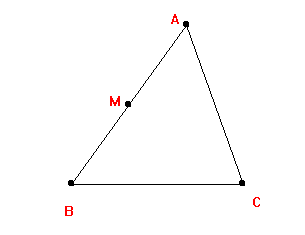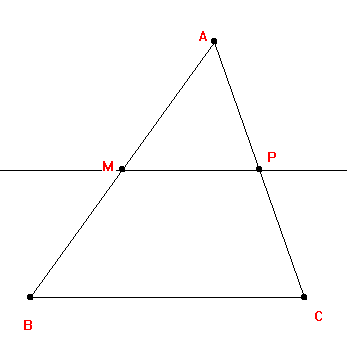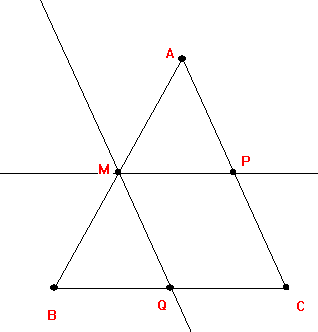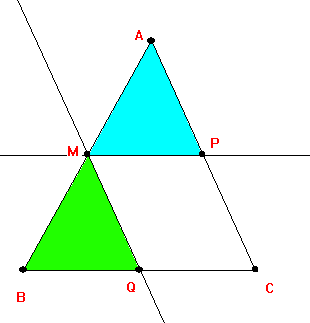Theorem: The line segment connecting the midpoints of  two sides of a triangle is parallel to the third side and is congruent to one half of the third side.
Proof:
 Consider the triangle ABC with the midpoint of  AB labelled M.Now construct a line through M parallel to BC. Label the point of intersection of this line with BC by P.Now construct a line through M parallel to AC. Label the point of intersection of this line with AC by QClaim: AMP is congruent to MBQ.  Why?• AM=BM [midpoint].
• < AMP = <MBQ [Corresponding angles for parallel lines cut by an transversal].
• <BQM=<QCP=<APM [Corresponding angles for parallel lines cut by an transversal].
• <BMQ=<MAP [When 2 pairs of corresponding angles are congruent in a triangle, the third pair is also congruent.]
• AMP is congruent to MBQ. [ASA]
Therefore: AP=MQ=PC and MP=BQ=QC.
Since midpoints are unique, and the lines connecting points are unique, the proposition is proven.

Corollary: In any triangle, the lines connecting the midpoints are parallel to the opposite sides and the four triangles created by these lines and the segments of the original triangle formed by the midpoints are all congruent..